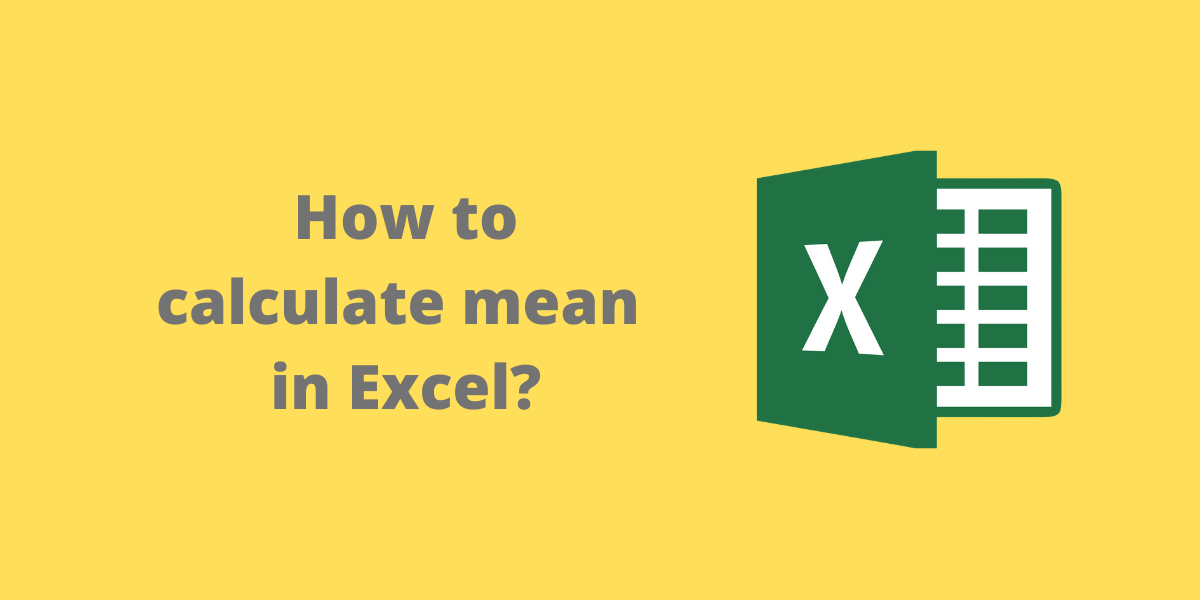# How to calculate mean in Excel? [Easy Step-by-Step Method]The mean is the average or the most common value in a set of numbers. In this article, we will learn how to calculate the mean in Excel.

## Steps to Calculate Mean in Excel

Let us consider the following dataset of a group of students with their marks:

We are interested in finding the mean of the marks scored by the students. To calculate the mean in Excel, we use an in-built AVERAGE function. The syntax of the AVERAGE function is =AVERAGE(start cell reference:end cell reference).

• Select the cell where you want to display the mean.
• Type the formula =AVERAGE( and select the data range containing the values for which mean value is sought.
• Finish the formula with ) and press the Enter key.

In this example, we enter the formula =AVERAGE(B2:B11) in cell B13, to get the mean of the marks obtained by students:

Note: The AVERAGE function ignores empty cells and cells that do not include any numbers.

## Conclusion

In this article, we learned how to calculate the mean in Excel using the AVERAGE formula.